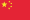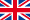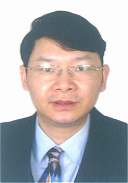����English
��ǰλ�ã���ҳ>>ʦ������>>���Ľ�ʦ
 ���� ������ ������ ����Ƽ ���ڻ� ��ά�� �� �� ���ʽ� �ΰ �۾��� ��Ц�� ������ ������ ��ż ���ȷ� ���� �ڴ���
 ��ְ���� �»� Peter Paule
 ������ ���� �� �� ��Э�� ���� ������ �軳ǫ ������ �α��� ������ ��ҫ�� �⻪�� ��� �� �� ��Ѷ �ź��� ������ ���
 ��ʦ ��Ӣ�� ������ ����� ���� ���� ���ľ� �λ��� κ�� л��ͥ ���� ����� ������
 ��ʿ�� ��� 2020-2023
 �칫�� ���� ���Ȼ ����� ��Сƽ������ ���� Ӧ����ѧ���Ľ�ʦ �� ҳ�� http://math.bnu.edu.cn/jzg/qtyg/115977.html �� ���� �� �䣺 wangfy@tju.edu.cn

�о�����

�������

����������

1983.09-1987.07������ʦ����ѧ ��ѧרҵ ����/ѧʿ

1987.09-1990.07������ʦ����ѧ ������������ͳ�� �о���/˶ʿ

1990.09-1993.07������ʦ����ѧ ������������ͳ�� �о���/��ʿ

����ר����

 F.-Y. Wang, Functional Inequalities, Markov Semigroups and Spectral Theory, Science Press. 2005.

 F.-Y. Wang, Harnack Inequality and Applications for Stochastic Partial Differential Equations, Springer, 2013

 F.-Y. Wang, Analysis of Diffusion Processes on Riemannian Manifolds, World Scientific, 2014.

�������ģ�

 F.-Y. Wang, Sharp explicit lower bounds of heat kernels, Ann. Probab. 25 (1997)

 F.-Y. Wang, Harnack inequalities for log-Sobolev functions and estimates of log-Sobolev constant, Ann. Probab. 27(1999)

 M. F. Chen and F.-Y. Wang, Cheeger��s inequalities for general symmetric forms and existence criterion for spectral gap, Ann. Probab. 28(2000)

 M. Cranston and F.-Y. Wang, Equivalence of coupling and shift-coupling, Ann. Probab. 28(2000)

 F.-Y. Wang, Gradient estimates of Dirichlet semigroups and applications to isoperimetric inequalities, Ann. Probab. 32 (2004)

 F.-Y. Wang, Harnack inequality and applications for stochastic generalized porous media equations, Ann. Probab. 35(2007)

 F.-Y. Wang, Log-Sobolev inequalities: different roles of Ric and Hess, Ann. Probab. 37(2009)

 F.-Y. Wang, Harnack inequality for SDE with multiplicative noise and extension to Neumann semigroup on non-convex manifolds, Ann. Probab. 39(2011)

 F.-Y. Wang, Integration by parts formula and shift Harnack inequality for stochastic equations, Ann. Probab. 42(2014)

 F.-Y. Wang Integrability Conditions for SDEs and Semi-linear SPDEs, Ann. Probab.. (accepted)

 M. F. Chen and F.-Y. Wang, On order-preservation and positive correlations for multidimensional diffusion processes, Probab. Theory Relat. Fields 95(1993)

 F.-Y. Wang, Application of coupling method to the Neumann eigenvalue problem, Probab. Theory Relat. Fields 98(1994)

 F.-Y. Wang, Estimates of the first Dirichlet eigenvalues by using diffusion processes, Probab. Theory Relat. Fields 101(1995)

 F.-Y. Wang, On estimation of logarithmic Sobolev constant and gradient estimates of heat semigroups, Probab. Theory Relat. Fields 108(1997)

 F.-Y. Wang, Logarithmic Sobolev inequalities on noncompact Riemannian manifolds, Probab. Theory Relat. Fields 109(1997)

 M. F. Chen, F.-Y. Wang, Estimation of the first eigenvalue of second order elliptic operators, J. Funct. Anal. 131(1995)

 M. F. Chen, F.-Y. Wang, Estimates of logarithmic Sobolev constant: an improvement of Bakry-Emery criterion, J. Funct. Anal. 144(1997)

 A. Thalmaier, F.-Y. Wang, Gradient estimates for harmonic functions on regular domains in Riemannian manifolds, J. Funct. Anal. 155(1998)

 F.-Y. Wang, Functional inequalities for empty essential spectrum, J. Funct. Anal. 170(2000)

 M. Rockner, F.-Y. Wang, Weak Poincare inequalities and convergence rates of Markov semigroups, J. Funct. Anal. 185(2001)

 F.-Y. Wang, Functional inequalities and spectrum estimates: the infinite measure case, J. Funct. Anal. 194 (2002)

 M. Rockner, F.-Y. Wang, Harnack and functional inequalities for generalized Mehler semigroups, J. Funct. Anal. 203 (2003)

 F.-Y. Wang, Probability distance inequalities on Riemannian manifolds and path spaces, J. Funct. Anal. 206 (2004)

 E. Priola and F.-Y. Wang, Gradient estimates for diffusion semigroups with singular coefficients, J. Funct. Anal. 236(2006)

 F.-Y. Wang, A Harnack-type inequality for Non-Symmetric Markov Semigroups, J. Funct. Anal . 239��2006��

 F.-Y. Wang, Second fundamental form and gradient of Neumann semigroups, J. Funct. Anal. 256(2009)

 G. Da Prato, M. Rockner, F.-Y. Wang, Singular stochastic equations on Hilbert spaces: Harnack inequalities for their transition semigroups, J. Funct. Anal. 257(2009)

 P. Cattiaux, A. Guillin, F.-Y. Wang, L. Wu, Lyapunov conditions for Super Poincar�� inequalities, J. Funct. Anal. 256(2009)

 S. Feng, W. Sun, F.-Y. Wang, F. Xu, Functional inequalities for the unlabeled two-parameter infinite-alleles diffusion, J. Funct. Anal. 260(2011)

 F.-Y.Wang, Criteria on spectral gap of Markov operators, J. Funct. Anal. 266(2014)

 F.-Y. Wang, L. Xu, X. Zhang, Gradient estimates for SDEs driven by multiplicative Levy noise, J. Funct. Anal.269(2015), 3195--3219.

 M. F. Chen, F.-Y. Wang, Estimation of spectral gap for elliptic operators, Trans. Amer. Math. Soc. 349:3(1997)

 V. I. Bogachev, M. Rockner, F.-Y. Wang, Elliptic equations for invariant measures on finite and infinite dimensional manifolds, J. Math. Pure Appl. 80(2001)

 D. Bakry, M. Ledoux and F.-Y. Wang, Perturbations of functional inequalities using growth conditions, J. Math. Pure Appl. 87(2007)

 F.-Y. Wang, From super Poincare to weighted log-Sobolev and entropy-cost inequalities, J. Math. Pure Appl. 90(2008)

 F.-Y. Wang, Harnack inequalities on manifolds with boundary and applications, F.-Y. Wang, Harnack inequalities on manifolds with boundary and applications, J. Math. Pures Appl. 94(2010)

 F.-Y. Wang, X. Zhang, Derivative formula and applications for degenerate diffusion semigroups, J. Math. Pures Appl. 99(2013)

 V.I. Bogachev, M. Rockner, M., F.-Y. Wang, Invariance implies Gibbsian: some new results, Comm. Math. Phys. 248 (2004)

 J. Ren, M. Rockner, F.-Y. Wang, Stochastic generalized porous media and fast-diffusion equations, J. Diff. Equations 238(2007)

 M. Rockner, F.-Y. Wang, Non-monotone stochastic generalized porous media equations, J. Diff. Equations 245(2008)

 A. Guillin, F.-Y. Wang, Degenerate Fokker-Planck Equations : Bismut Formula, Gradient Estimate and Harnack Inequality, J. Diff. Equations 253(2012)

 F.-Y. Wang, Gradient Estimates and Applications for SDEs in Hilbert Space with Multiplicative Noise and Dini Continuous Drift, J. Diff. Equations 260 (2016)

 F.-Y. Wang, Log-Sobolev inequality on non-convex manifolds, Advances in Math. 222(2009)